## #8 2011-03-16 16:00:30

YourLocalBlockLib
Scratcher
Registered: 2011-03-10
Posts: 100+

### Re: ITopic: Welcome to your local block library!

Operators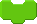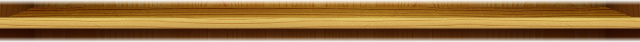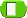Shared by Zorket.
Blockspec:

('report %s' #r #report: 'hello')

Code:

report: t1
^ t1

What it does:
Reports it's contents. Useful for putting text in areas where it normally can't go. (Such as the [go to %m] block.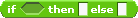Shared by Zorket, optimised by nXIII
Blockspec:

('if %b then %s else %s' #r #if:then:else:)

Code:

if: t1 then: t2 else: t3
t1 ifTrue: [^ t2].
^ t3

What it does:
Reports the first string argument if the boolean is true, the second if it's false.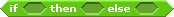Shared by Zorket
Blockspec

('if %b then %b else %b' #b #if:then:else:)

Code:

if: t1 then: t2 else: t3
t1 ifTrue: [^ t2].
^ t3

What it does
Reports the truth of the first or second boolean argument depending on the truth of the first.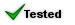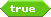Shared by Zorket
Blockspec

('true' #b #reportTrue)

Code:

reportTrue
^ true

What it does
Reports true.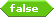Shared by Zorket
Blockspec

('false' #b #reportFalse)

Code:

reportFalse
^ false

What it does
Reports false.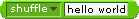Shared by Zorket
Blockspec

('%T %s' #r #do:to:)

#### Code:

```do: t1 to: t2
t1 = 'reverse' ifTrue: [^ t2 reversed].
t1 = 'shuffle' ifTrue: [^ t2 shuffled].
t1 = 'uppercase' ifTrue: [^ t2 asUppercase].
t1 = 'lowercase' ifTrue: [^ t2 asLowercase].
t1 = 'report' ifTrue: [^ t2].```

Scratch-Blocks> CommandBlockMorph> private> uncoloredArgMorphFor: add a strip:

#### Code:

```\$T = t2 ifTrue: [^ ChoiceOrExpressionArgMorph new getOptionsSelector: #mixingNames;
choice: 'reverse'].```

Scratch objects> scriptableScratchMorph> otherOps:

mixingNames
^ #('lowercase' 'uppercase' 'reverse' 'shuffle' 'report' )

What this block does:
Does the operation on the specified string.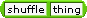Shared by poemon1, based on the above block
Blockspec:

('%s %s' #r #do:to: 'shuffle' 'thing')

Code:
Same as above block.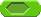Shared by Hardmath123.
Blockspec:

('%b' #r #booleanString:)

Code:

booleanString: t1
^ t1

What it does:
Allows you to drop booleans into reporter spaces!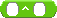Shared by Hardmath123.
Blockspec:

('%n ^ %n' #r #x:expy: 2 2)

Code:

x: t1 expy: t2
^ t1 raisedTo: t2

What it does:
Reports n1 to the power of n2.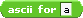Shared by Pecola1.
Blockspec:

('ascii for %s' #r #asciiCodeOf:)

Code:

asciiCodeOf: aString
| str |
str _ aString asMacRoman.
str size = 1 ifFalse: [^ 0].
^ str first asciiValue

What it does:
Reports the ASCII code for an entered character.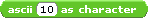Shared by Pecola1.
Blockspec:

('ascii %n as character' #r #asciiLetter:)

Code:

asciiLetter: anInteger
| code |
code _ anInteger asNumberNoError.
^ String with: (Character value: code)

What it does:
Reports character the entered ASCII code stands for.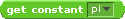Shared by Hardmath123, improved by Baderous
Blockspec:

('%G' #r #getConstant:)

Code:

getConstant: t1
t1 = 'pi' ifTrue: [^ float pi].
t1 = 'phi' ifTrue: [^ float phi].
t1 = 'e' ifTrue: [^ float e].
t1 = 'sqrt 2' ifTrue: [^ 2 sqrt].
^ self error

Scratch-Objects»ScriptableScratchMorph»sensing

constants
^ #('pi' 'phi' 'e' 'sqrt 2' )

Scratch-Blocks»CommandBlockMorph»-- all --»uncoloredArgMorphFor: add a line:

\$G = t2 ifTrue: [^ ChoiceOrExpressionArgMorph new getOptionsSelector: #constants;
choice: 'pi'].

What it does:
Reports the selected constant.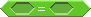Shared by Hardmath123.
Blockspec:

('%b=%b' #b #a:equalsB:)

Code:

a: t1 equalsB: t2
t1 = t2 ifTrue [^true].
^false.

What it does:
Reports whether or not the two booleans are equal.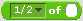Shared by ESN.
What it does:
Reports a fraction of a number from a dropdown.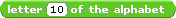Shared by Zorket.
Blockspec

('letter %n of the alphabet' #r #letter:)

Code:

#### Code:

```letter: t1
t1 = 1 ifTrue: [^ 'a'].
t1 = 2 ifTrue: [^ 'b'].
t1 = 3 ifTrue: [^ 'c'].
t1 = 4 ifTrue: [^ 'd'].
t1 = 5 ifTrue: [^ 'e'].
t1 = 6 ifTrue: [^ 'f'].
t1 = 7 ifTrue: [^ 'g'].
t1 = 8 ifTrue: [^ 'h'].
t1 = 9 ifTrue: [^ 'i'].
t1 = 10 ifTrue: [^ 'j'].
t1 = 11 ifTrue: [^ 'k'].
t1 = 12 ifTrue: [^ 'l'].
t1 = 13 ifTrue: [^ 'm'].
t1 = 14 ifTrue: [^ 'n'].
t1 = 15 ifTrue: [^ 'o'].
t1 = 16 ifTrue: [^ 'p'].
t1 = 17 ifTrue: [^ 'q'].
t1 = 18 ifTrue: [^ 'r'].
t1 = 19 ifTrue: [^ 's'].
t1 = 20 ifTrue: [^ 't'].
t1 = 21 ifTrue: [^ 'u'].
t1 = 22 ifTrue: [^ 'v'].
t1 = 23 ifTrue: [^ 'w'].
t1 = 24 ifTrue: [^ 'x'].
t1 = 25 ifTrue: [^ 'y'].
t1 = 26 ifTrue: [^ 'z'].
^ self error```

What it does:
Gives you the letter from the alphabet corresponding to the number you enter.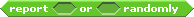Shared by Pecola1.
Blockspec:

('report %b or %b randomly' #b #booleanRandom:Or:)

Code:

booleanRandom: t1 Or: t2
| t3 |
t3 _ self randomFrom: 0 to: 1.
t3 = 1 ifTrue: [^ t1].
^ t2

What it does:
Reports either of the booleans randomly.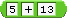Shared by Zorket.
Blockspec:

('%n %s %n' #r #workOut:with:to:)

Code:

workOut: t1 with: t2 to: t3
t2 = '+' ifTrue: [^ t1 + t3].
t2 = '-' ifTrue: [^ t1 - t3].
t2 = '*' ifTrue: [^ t1 * t3].
t2 = '/' ifTrue: [^t1 / t3].
^ 'Error!'

What it does:
Designed to replaces the +, -, * and / reporter blocks.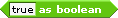Shared by Pecola1.
Blockspec:

('%s as boolean' #b #stringAsBoolean:)

Code:

stringAsBoolean: t1
| t2 |
t2 _ t1 asString.
(t2 = '0'
or: [t2 = '' or: [t2 = 'false']])
ifTrue: [^ false].
^ true

What it does:
Reports false if the string is blank, 0, or "false," otherwise reports true.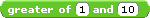Shared by Hardmath123.
Blockspec:

('greater of %n and %n' #r #greater:and:)

Code:

greater: t1 and: t2
t1 > t2 ifTrue: [^ t1].
^ t2

What it does:
Reports the greater of the two values.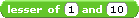Shared by Hardmath123.
Blockspec:

('lesser of %n and %n' #r #greater:and:)

Code:

lesser: t1 and: t2
t1 < t2 ifTrue: [^ t1].
^ t2

What it does:
Reports the lesser of the two values.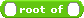Shared by Hardmath123
Blockspec:

('%n root of %n' #r #root:of: 2 64)

Code:

root: t1 of: t2
t2 > 0 ifTrue: [^ t2 raisedTo: 1 / t1].
^ t2 * -1 raisedTo: 1 / t1

What it does:
Reports the root of a number; i.e. the inserts 2 and 64 would be 8, 8 is the square root of 64.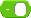Shared by hello12345678910
Blockspec:

('-%n' #r #negation:)

Code:

negation: t1
^ t1 * -1

What it does:
Reports the negative of a number.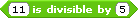Shared by Hardmath123
Blockspec:

('%n is divisible by %n' #b #test:div: 11 5)

Code:

test: t1 div: t2
^ t1 / t2 = (t1 / t2) rounded

What it does:
Does a divisibility test.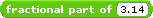Shared by Hardmath123
Blockspec:

('fractional part of %n' #r #fractional: 3.14)

Code:

fractional: t1
^ t1 - (t1 - 0.5) rounded

What it does:
Reports the digits after the decimal point.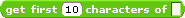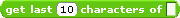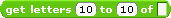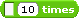Shared by Hardmath123

These blocks all rely on one-another's code so you need to install them all at once.

Blockspecs:

('Get the first %n letters of %s' #r #getNum:string: 3 #'scratch')

('Get the last %n letters of %s' #r #getLastNum:string: 3 #'scratch')

('Get letters %n to %n of %s' #r #getLetter:to:of: 1 4 #'scratch')

('%s %n times' #r #string:times: 'scratch ' '2')

Codes:

getNum: t1 string: t2
| t3 t4 |
t1 = 1 ifTrue: [^ self letter: 1 of: t2].
t3 _ self getNum: t1 - 1 string: t2.
t4 _ self letter: t1 of: t2.
^ t3, t4

getLastNum: t1 string: t2
^ (self getNum: t1 string: t2 reversed) reversed

getLetter: t1 to: t2 of: t3
| t4 t5 |
t4 _ self getNum: t3 size + 1 - t2 string: t3.
t5 _ self getLastNum: t4 size + 1 - t1 string: t4.
^ t5

string: t1 times: t2
t2 = 1 ifTrue: [^ t1].
^ (self string: t1 times: t2 - 1)
, t1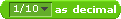Shared by jslomba and improved by Scimonster

This block requires the ([fraction] of ( ) ) block above by ESN.

Blockspec:

('%z as decimal' #r #decimalOf:)

Code:

decimalOf: t1
'1/2' = t1 ifTrue: [^ 1/2].
'1/3' = t1 ifTrue: [^ 1/3].
'1/4' = t1 ifTrue: [^ 1/4].
'1/5' = t1 ifTrue: [^ 1/5].
'1/6' = t1 ifTrue: [^ 1/6].
'1/7' = t1 ifTrue: [^ 1/7].
'1/8' = t1 ifTrue: [^ 1/8].
'1/9' = t1 ifTrue: [^ 1/9].
'1/10' = t1 ifTrue: [^ 1/10]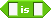Shared by Scimonster
Blockspec:

('%s is %s' #b #case:sensitive:)

Code:

case: t1 sensitive: t2
t1 = t2 ifTrue: [^ true].
^ false

What this block does:
Is a case-sensitive <[] = []> block.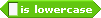Shared by Scimonster
Blockspec:

('%s is lowercase' #b #isLowercase:)

Code:

isLowercase: t1
t1 = '' ifTrue: [^ false].
t1 = t1 asLowercase ifTrue: [^ true].
^ false

What this block does:
Reports whether or not the inputted text is lowercase.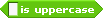Shared by Scimonster
Blockspec:

('%s is uppercase' #b #isUppercase:)

Code:

isUppercase: t1
t1 = '' ifTrue: [^ false].
t1 = t1 asUppercase ifTrue: [^ true].
^ false

What this block does:
Reports whether or not the inputted text is uppercase.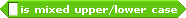Shared by Scimonster
Blockspec:

('%s is uppercase' #b #isMixed:)

Code:

isMixed: t1
t1 = t1 asLowercase ifTrue: [^ false].
t1 = t1 asUppercase ifTrue: [^ false].
^ true

What this block does:
Reports whether or not the inputted text is mixed uppercase and lowercase.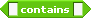Shared by LS97
Blockspec:

('%s contains %s' #b #if:contains:)

Code:

if: t1 contains: t2
(t1 findString: t2)
> 0 ifTrue: [^ true].
^ false

What this block does:
Reports whether a given string contains another.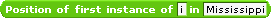Shared by Hardmath123
Blockspec:

('Position of first instance of %s in %s' #r #index:of: 'i' 'Mississippi')

Code:

index: t2 of: t1
^t1 findString: t2

What this block does:
Finds the first instance of a string in another.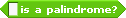Shared by Hardmath123
Blockspec:

('%s is a palindrome?' #b #palindrome:)

Code:

palindrome: i1
| t1 |

t1_i1.
t1_ t1 asString reversed.
t1 = i1 ifTrue: [^ true].
^ false

What this block does:
Reports whether or not the block is a palindrome.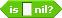Shared by jsombla, improved by Scimonster
Blockspec:

('is %s nil?' #b #checkNil:)

Code:

checkNil: str
^ str isNil

What this block does:
Checks if the content is nil.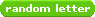Shared by Greenatic
Blockspec:

('random letter' #r #randomLetter)

Code:

#### Code:

```randomLetter
| t1 |
t1 _ self randomFrom: 1 to: 26.
t1 = 1 ifTrue: [^ 'a'].
t1 = 2 ifTrue: [^ 'b'].
t1 = 3 ifTrue: [^ 'c'].
t1 = 4 ifTrue: [^ 'd'].
t1 = 5 ifTrue: [^ 'e'].
t1 = 6 ifTrue: [^ 'f'].
t1 = 7 ifTrue: [^ 'g'].
t1 = 8 ifTrue: [^ 'h'].
t1 = 9 ifTrue: [^ 'i'].
t1 = 10 ifTrue: [^ 'j'].
t1 = 11 ifTrue: [^ 'k'].
t1 = 12 ifTrue: [^ 'l'].
t1 = 13 ifTrue: [^ 'm'].
t1 = 14 ifTrue: [^ 'n'].
t1 = 15 ifTrue: [^ 'o'].
t1 = 16 ifTrue: [^ 'p'].
t1 = 17 ifTrue: [^ 'q'].
t1 = 18 ifTrue: [^ 'r'].
t1 = 19 ifTrue: [^ 's'].
t1 = 20 ifTrue: [^ 't'].
t1 = 21 ifTrue: [^ 'u'].
t1 = 22 ifTrue: [^ 'v'].
t1 = 23 ifTrue: [^ 'w'].
t1 = 24 ifTrue: [^ 'x'].
t1 = 25 ifTrue: [^ 'y'].
t1 = 26 ifTrue: [^ 'z']```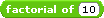Shared by Greenatic
Blockspec:

('factorial of %n' #r #Factorial:)

Code:

Factorial: t1
t1 isInf ifTrue:[^0].
t1 isNaN ifTrue:[^0].
t1store _ t1.
t1 = 0 ifTrue: [^ 1].
t1 < 0 ifTrue: [^ 0].
t1 isInf ifTrue: [^ 0].
t1 isNaN ifTrue: [^ 0].
[t1store > 1]
whileTrue:
t1store _ t1store - 1].

What this block does:
Reports the factorial of a number.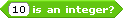Shared by Greenatic, improved by Scimonster
Blockspec:

('%n is an integer?' #b #isInteger:)

Code:

isInteger: t1
^ t1 = t1 rounded

What this block does:
Reports whether the number is an integer.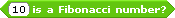Shared by Greenatic
Blockspec:

('%n is a Fibonacci number?' #b #IsFibonacci:)

Code:

IsFibonacci: t1
| fib1 fib2 oldfib2 fib3 oldfib3 |
t1 isInf ifTrue:[^false].
t1 isNaN ifTrue:[^false].
t1 < 0 ifTrue: [^ false].
t1 = 0 ifTrue: [^ true].
t1 = 1 ifTrue: [^ true].
fib1 _ 0.
fib2 _ 1.
oldfib2 _ fib2.
fib3 _ fib1 + fib2.
oldfib3 _ fib3.
[t1 > fib3]
whileTrue:
[fib3 _ fib1 + fib2.
fib2 _ oldfib3.
fib1 _ oldfib2.
oldfib3 _ fib3.
oldfib2 _ fib2].
t1 = fib3 ifTrue: [^ true].
t1 = fib3 ifFalse: [^ false]

What this block does:
Reports if the block is a Fibonacci number.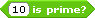Shared by Greenatic
Blockspec:

('%n is prime?' #b #IsPrime:)

Code:

IsPrime: t1
| divisor factor |
t1 isInf ifTrue: [^ false].
t1 isNaN ifTrue: [^ false].
t1 < 1 ifTrue: [^ false].
t1 = 1 ifTrue: [^ false].
t1 = t1 rounded ifFalse:[^false].
divisor _ 2.
[divisor > (0.5 * t1)]
whileFalse:
[factor _ t1 / divisor.
factor = factor rounded ifTrue: [^false].
divisor _ divisor + 1].
^ true

What this block does:
Reports if the number is prime.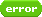Shared by Lots of People
Blockspec:

('error' #r #error)

Code:
No code needed
What this block does:
Reports an error.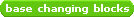Shared by Greenatic
Click the image for the codes. (13 blocks)
What these blocks do:
Let's you do arithmetic in any base from -36 to 36 (except for base -1 and base 0) and change between any of those bases.^ Back to Scratch Blocks
^ Back to Contents

Last edited by YourLocalBlockLib (2011-09-09 04:54:37)

Offline